# More on DeepSig’s RML Data Sets

The second DeepSig data set I analyze: SNR problems and strange PSDs.

I presented an analysis of one of DeepSig’s earlier modulation-recognition data sets (RML2016.10a.tar.bz2) in the post on All BPSK Signals. There we saw several flaws in the data set as well as curiosities. Most notably, the signals in the data set labeled as analog amplitude-modulated single sideband (AM-SSB) were absent: these signals were only noise. DeepSig has several other data sets on offer at the time of this writing:

In this post, I’ll present a few thoughts and results for the “Larger Version” of RML2016.10a.tar.bz2, which is called RML2016.10b.tar.bz2. This is a good post to offer because it is coherent with the first RML post, but also because more papers are being published that use the RML 10b data set, and of course more such papers are in review. Maybe the offered analysis here will help reviewers to better understand and critique the machine-learning papers. The latter do not ever contain any side analysis or validation of the RML data sets (let me know if you find one that does in the Comments below), so we can’t rely on the machine learners to assess their inputs. (Update: I analyze a third DeepSig data set here. And a fourth and final one here.)

As a preview, the data set contains two identifiers for each short (128 sample) I/Q signal: the modulation type and an SNR parameter. The SNR parameter does not correspond to any definition I know, and if you use it as if it were the SNR of the signal snippet, you’ll be off by tens of dB–the signals are much stronger than indicated by the parameter. I see papers that are using it as if was the SNR, and this makes the machine-learning algorithm results look much much better than they really are.

So let’s take a look.

I wrote a python program to extract the first 1000 instances of each signal and SNR-parameter combination, and then estimated their power spectral densities. I plot the 1000 PSDs for each signal/SNR-parameter on a single set of axes. The python program is similar to the one in All BPSK Signals, and runs to completion without any error or discrepancy I can see, so I’m confident (but not completely certain) that the data is correctly extracted.

To make this post cleaner, I also arrange the PSD plots for a single signal in a movie file, so you can get a feeling for how the “spectrum analyzer look” of the signal varies as the SNR parameter changes. For example, here is the movie for BPSK:

The power-spectrum estimation method is the frequency-smoothing method and the width of the frequency-smoothing window is 10% of the sampling rate.

A couple things stand out about the BPSK movie. First, as in the RML2016.10a.tar.bz2 data set, the PSDs for the SNR parameter value of -2 show two very different kinds of signals. About half of them show an obvious signal in noise and half appear to be noise only: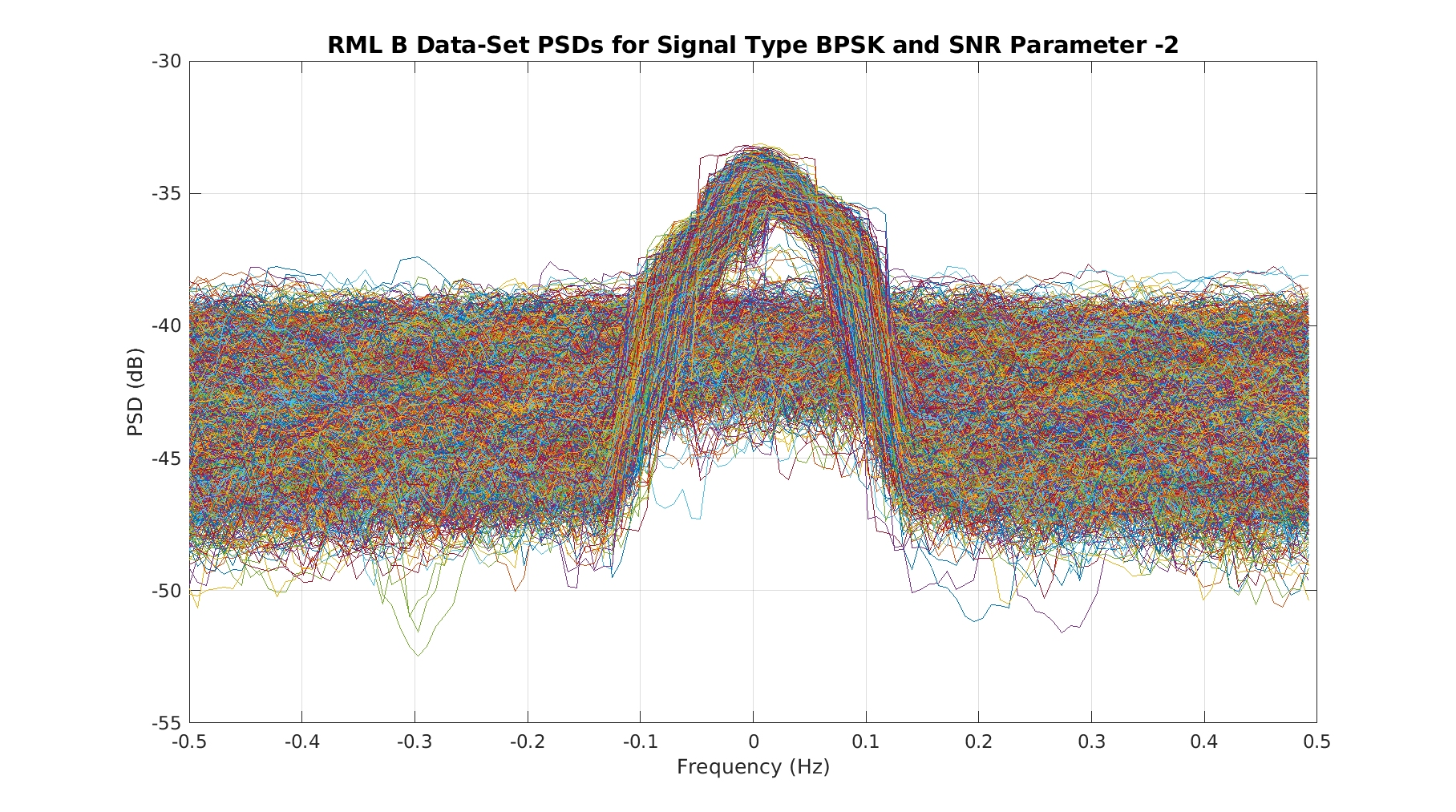Figure 1. CSP-Blog analysis of BPSK signals extracted from the RML-B 2016 publicly available data set for SNR parameter equal to -2. 1000 signals are extracted and processed. Note the presence of two kinds of PSD traces: signal-plus-noise and noise-only.

The BPSK PSDs for the next SNR parameter, 0, show much less evidence of this bifurcation: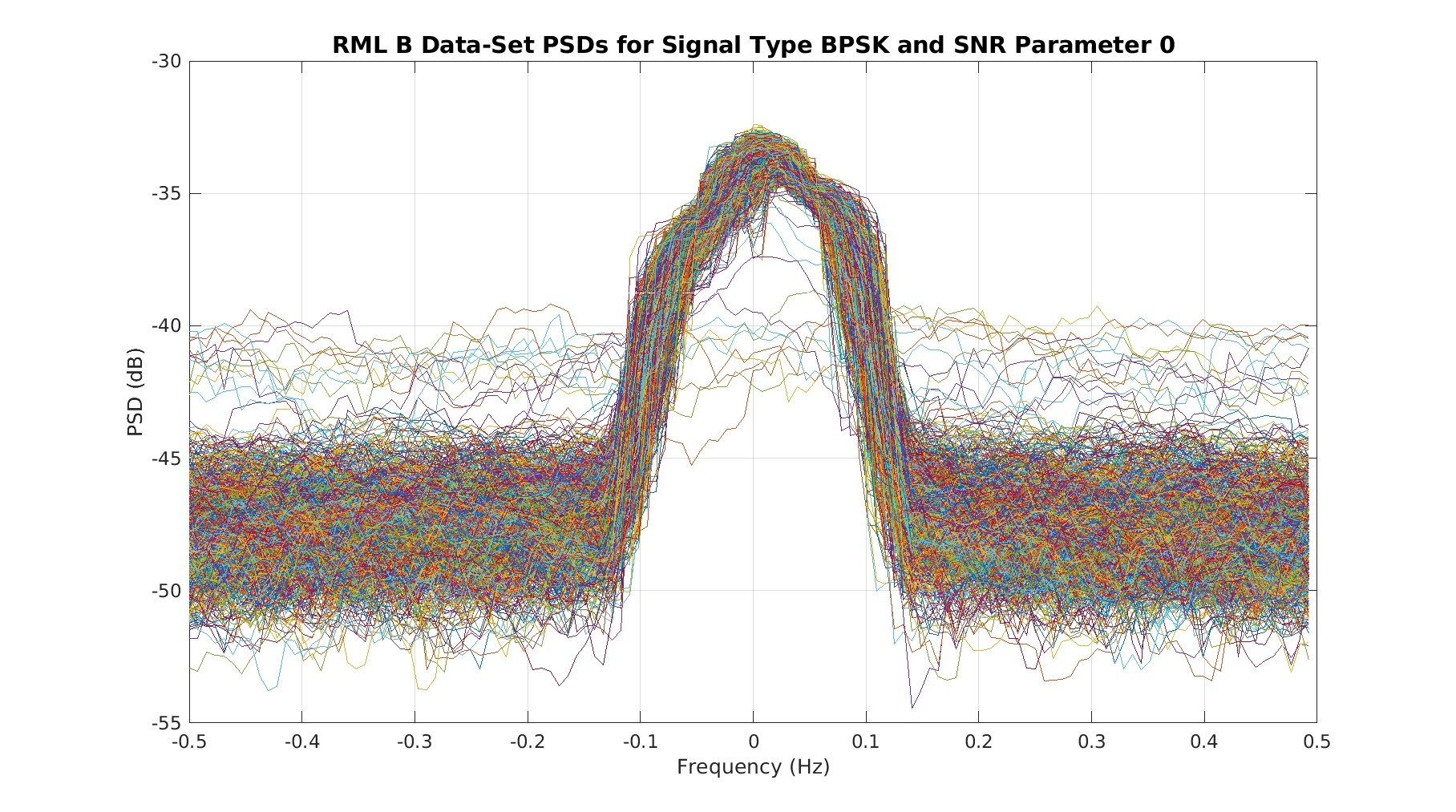Figure 2. CSP-Blog analysis of BPSK signals extracted from the RML-B 2016 publicly available data set for SNR parameter equal to 0. 1000 signals are extracted and processed.

The same is true for the remaining nine signal types in the data set, although the level of the noise-only traces relative to the noise floor for the signal-plus-noise traces is variable. Here is 64QAM:Figure 3. CSP-Blog analysis of 64-QAM signals extracted from the RML-B 2016 publicly available data set for SNR parameter equal to -2. 1000 signals are extracted and processed. Note the presence of two kinds of PSD traces: signal-plus-noise and noise-only. Also note that the noise floor for the signal-plus-noise traces is not the same as that for BPSK.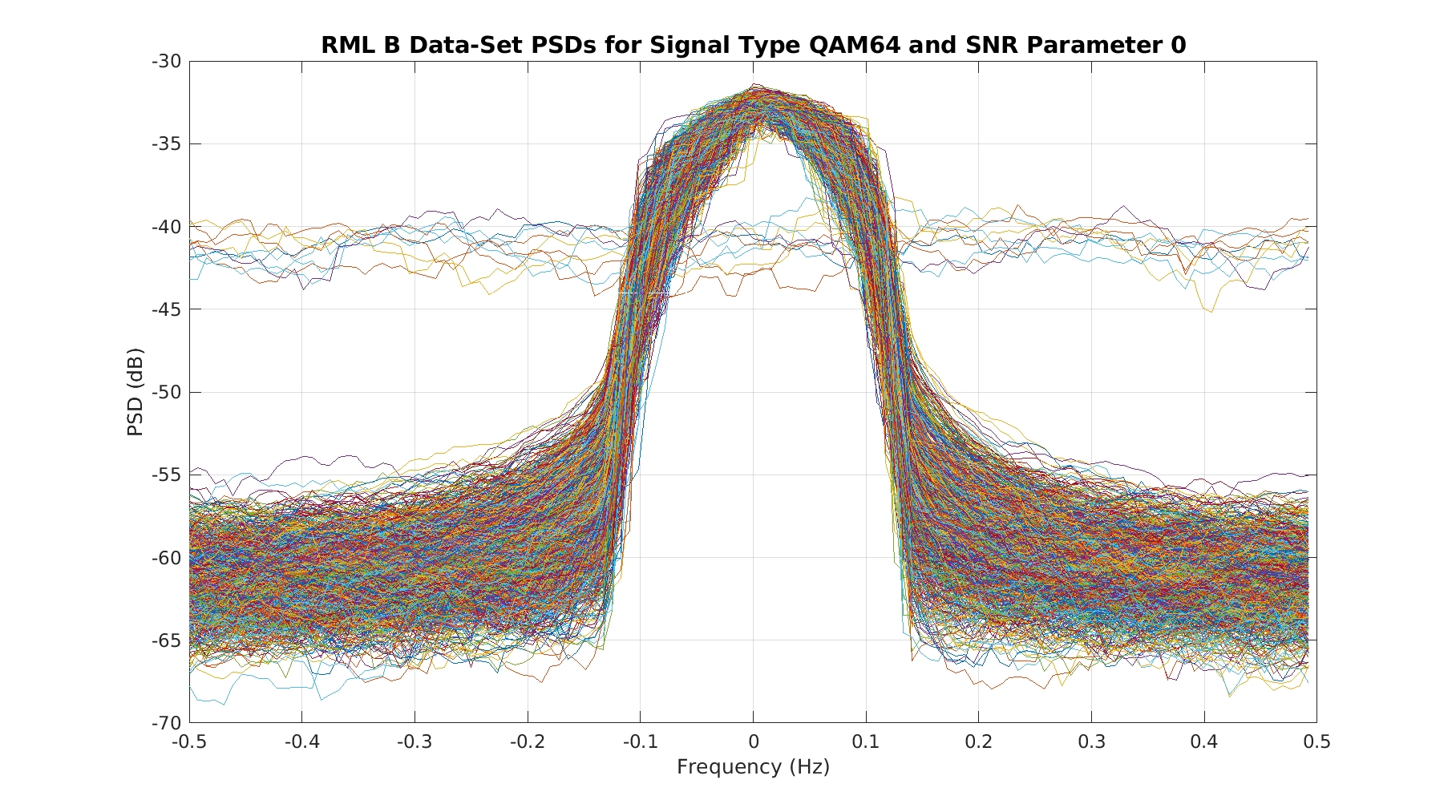Figure 4. CSP-Blog analysis of 64-QAM signals extracted from the RML-B 2016 publicly available data set for SNR parameter equal to 0. 1000 signals are extracted and processed.

Not all signal types show this behavior for the SNR parameter of 0. Some of them show the bifurcated PSD effect only for SNR parameter -2. For example, here are the results for CPFSK (what kind of CPFSK [My Papers ]? …who knows.):Figure 5. CSP-Blog analysis of CPFSK signals extracted from the RML-B 2016 publicly available data set for SNR parameter equal to -2. 1000 signals are extracted and processed. Note the presence of two kinds of PSD traces: signal-plus-noise and noise-only.Figure 6. CSP-Blog analysis of CPFSK signals extracted from the RML-B 2016 publicly available data set for SNR parameter equal to 0. 1000 signals are extracted and processed.

I placed the PSD movies for the remaining nine signal types at the end of the post.

Let’s turn to the relationship between the SNR parameter embedded in the signal archive and the actual SNR as determined through spectral analysis.

As a baseline, I created CSP-Blog RML-B-like BPSK and QPSK signals using eight samples per symbol, square-root raised-cosine pulses with excess bandwidth of 0.35, and carrier offset of zero. First I specified an inband SNR of 18 dB by choosing the signal power as unity and the noise power as 0.1 (-10 dB). The occupied bandwidth of the signal is about 0.17 (= (1+0.35)/8). So the inband SNR is calculated as$S_i = \frac{P_s}{BN_0} = \frac{1}{(0.1)(0.17)} = 17.7 \mbox{\rm \ dB} \hfill (1)$

On the other hand, many engineers use total SNR instead of inband SNR. The total SNR is simply the ratio of the signal power to the total noise power in the sampling bandwidth:$S_t = \frac{P_s}{N_0} = \frac{1}{0.1} = 10 \mbox{\rm \ dB} \hfill (2)$

I then computed the PSD estimates for 1000 blocks of data, each 128 samples long to be consistent with RML-B, using an FSM identical to that I applied to the RML-B data above. Here is what the CSP-Blog RML-B-like BPSK and QPSK PSD plots look like:Figure 7. CSP-Blog-generated RML-B-like BPSK signals for an inband-SNR of about 18 dB. The PSD shape is consistent with expectations based on theory and also is similar to the signal-plus-noise PSD traces of the RML-B data set.Figure 8. CSP-Blog-generated RML-B-like QPSK signals for an inband-SNR of about 18 dB. The PSD shape is consistent with expectations based on theory and also is similar to the signal-plus-noise PSD traces of the RML-B data set.

As you can see, the noise floor is variable but is centered at the correct value of -10 dB. The peak of the signal PSD is at about 8 dB, so a crude estimate of the signal power can be had (neglecting the inband noise-power contribution) by integrating the PSD over the signal bandwidth and approximating the spectrum by a rectangle:$\hat{P}_s = 10^{8/10} * 0.17 \approx 1.07 \hfill (3)$

Taking into account the small amount of inband noise yields 1.05, a bit closer to the known value of 1.

So everything is consistent and explicable with respect to the CSP-Blog RML-B-like signals.

If the RML-B SNR parameter indicates inband SNR, we would expect the RML-B PSDs for parameter 18 to be consistent with the CSP-Blog RML-B-like PSDs above. Here are the RML-B BPSK and QPSK PSDs for SNR parameter 18:Figure 9. RML-B PSD estimates for BPSK signals with SNR parameter of 18. Note the difference between the noise-floor variation here and the variation in Figure 7.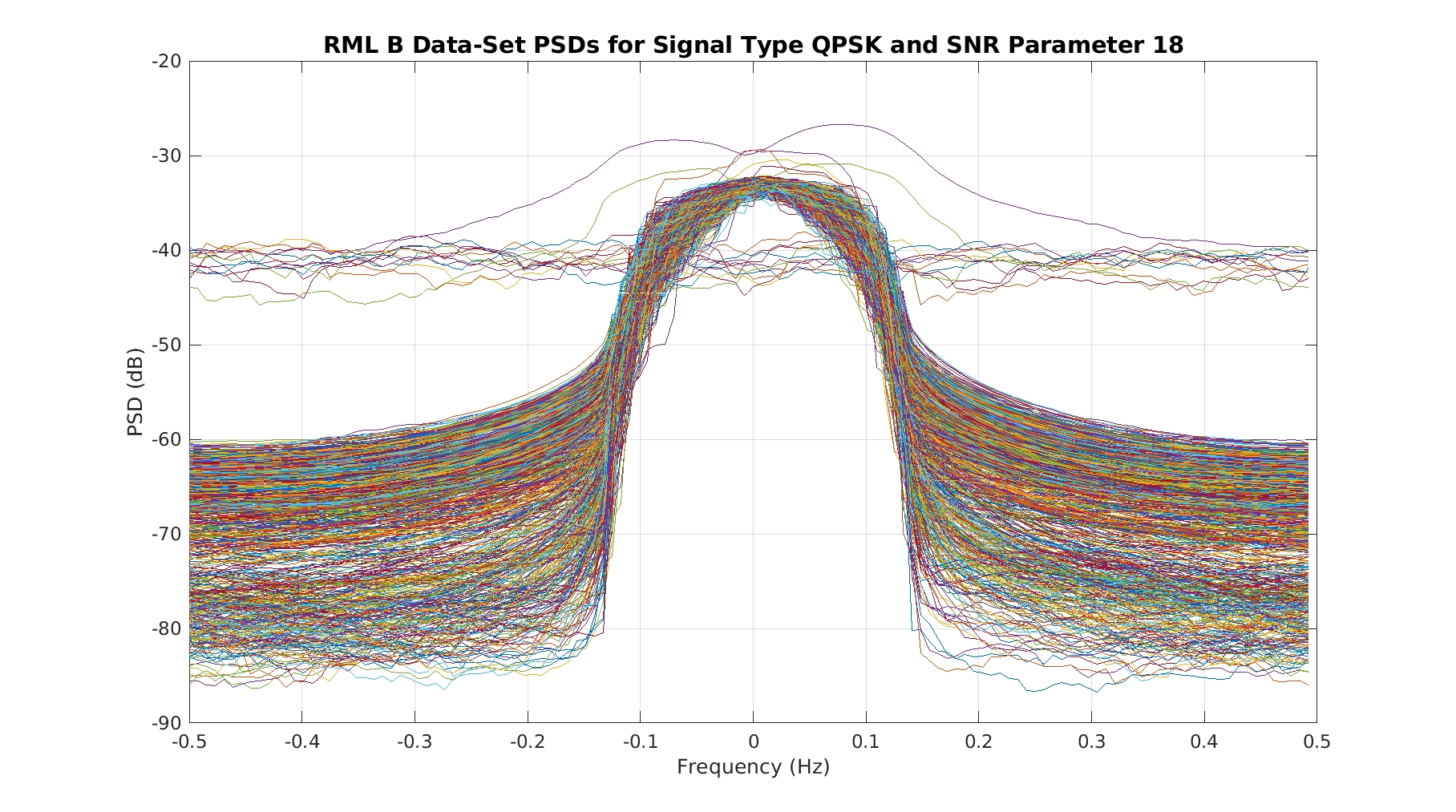Figure 10. RML-B PSD estimates for QPSK signals with SNR parameter of 18. Note the difference between the noise-floor variation here and the variation in Figure 8.

The first thing to notice is that the variability in the noise floor is much bigger than in the CSP-Blog RML-B-like signal PSDs. The variability in the latter is about 5 dB, that in the former is 25 dB. So there is no single SNR for these signals. When I create CSP-Blog RML-B-like signals with inband SNR of about 40 dB, I get an increase in the noise-floor variation:Figure 11. CSP-Blog-generated RML-B-like BPSK signals for an inband-SNR of about 40 dB. Compare to Figures 7 (CSP-Blog BPSK for inband SNR of 18 dB) and Figure 9 (RML-B BPSK for SNR parameter 18).

For 40 dB SNR, the variation increased to about 10 dB, still far short of the variation observed in the SNR-parameter-18 RML-B PSDs.

The second thing to notice is that the difference between the peak of the PSD and the average noise floor is huge in the RML-B PSDs. It is approximately -33 – (-73) = 40 dB. In the CSP-Blog RML-B-like PSD for inband SNR of 17.5 dB it is 8 – (-10) = 18 dB.

Let’s compute the SNR for the RML-B BPSK signal set directly. Staying with SNR parameter 18, the peak of the PSD is at about -33 dB (on the average), which is equal to about 0.0005 in non-decibel (Watts/Hz) units. The average noise floor has value of about -73 dB, or 5.0e-8 in non-decibel units. So we can compute the inband SNR as follows (see above for the same calculation for a CSP-Blog RML-B-like signal),$S_i \approx \frac{0.0005*0.17}{0.00000005*0.17} = 10000 = 40 \mbox{\rm \ dB} \hfill (4)$

Note that many of the signals will have an even larger SNR because we used the middle noise floor out of all observed noise floor values ranging from -60 dB to about -85 dB.

The total SNR is calculated as$S_t \approx \frac{0.0005*0.17}{0.00000005*1} = 1700 = 32 \mbox{\rm \ dB} \hfill (5)$

Let’s now look at the SNRs for the RML-B SNR parameter of 0. Here are four different signals’ PSDs for SNR parameter of 0:Figure 13. RML-B PSDs for BPSK and SNR parameter of 0. 1000 signals are extracted and processed. Note here that the variability in the noise floor and the difference between the PSD peak and the average noise floor closely mirrors that of the CSP-Blog BPSK signal in Figure 7, which has an inband SNR of 18 dB, not 0 dB.Figure 14. RML-B PSDs for PAM4 and SNR parameter of 0.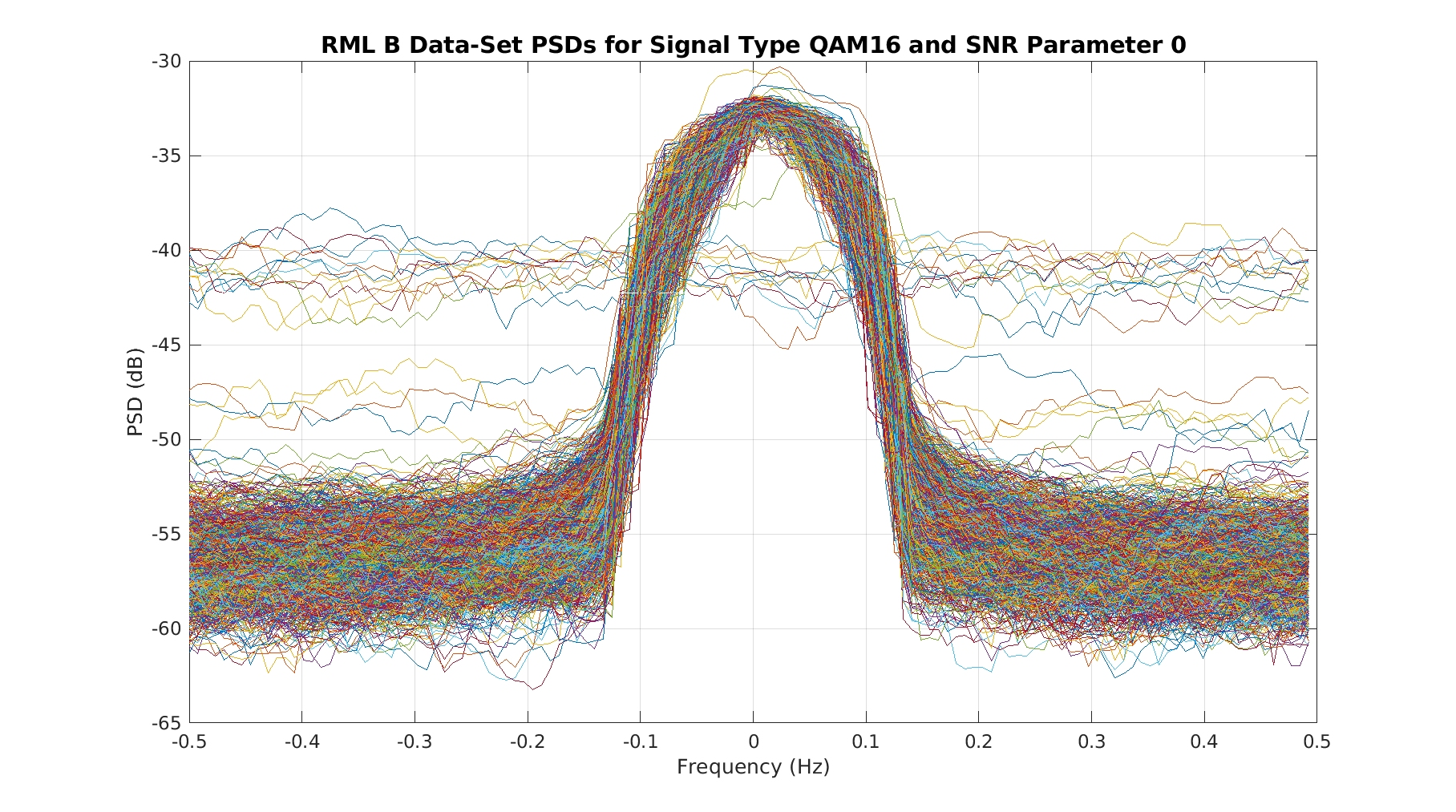Figure 15. RML-B PSDs for QAM16 and SNR parameter of 0.Figure 16. RML-B PSDs for QAM64 and SNR parameter of 0.

Using our approximate SNR calculation method, we find the inband and total SNRs for SNR parameter of 0 as indicated in Table 1.

None of the calculated SNRs is close to the SNR parameter of 0. Moreover, it appears that the signal power is the same, or quite similar, for all PSD traces where a signal appears to exist, but that the noise floor varies from signal type to signal type even for the same SNR parameter.

The more I look at the details of the DeepSig RML-B data set, the more I think that I must be missing something or I am simply in error. (“Though … I am unconscious of intentional error, I am nevertheless too sensible of my defects not to think it probable that I may have committed many errors.” G. Washington/Lin-Manuel Miranda) But there are, as with the RML2016.10a.tar.bz2 data set, many aspects of the extracted signals that are self-consistent and consistent with DeepSig’s published descriptions of the signals. Here are some things that give me confidence that the signals are properly extracted from the posted archive:

1. The observed signal strength does increase as the SNR parameter increases from -20 to +18.
2. When the signal is present (which is most of the time), the PSK, QAM, and PAM signal types show a PSD that is consistent with the stated symbol rate of 1/8 and a square-root raised-cosine pulse function with excess bandwidth of 35%.
3. The CPFSK signal PSD is somewhat different from the PSK/QAM/PAM PSDs, and is a plausible CPM PSD.
4. The AM and FM PSDs are consistent with a signal possessing a strong inband additive sine-wave component, as AM and FM signals sometimes do.
5. Not all of the signal types exhibit the ‘floating noise floor’ traces like the PSK, QAM, and PAM types do. The AM-DSB, WBFM, CPFSK, and GFSK signal types do not (although I’ve not checked each and every 1024-sample instance of every signal type). This suggests that the PSK, QAM, and PAM signal generators behind the data set are either flawed or those signal types sometimes present very strange PSDs for these very short signal durations (these were not observed in my generation of RML-B-like BPSK and QPSK). That these three types share a common flaw is plausible because they are really just variations on a single theme: linear complex-valued pulse-amplitude-modulated signals.
6. I do not observe any mixtures of different types of signal spectra, such as some traces obviously being PSK/QAM and some obviously being AM-DSB.

The DeepSig Downloads page indicates that the RML2016.10b.tar.bz2 is a “larger version” of the RML2016.10a.tar.bz2 data set “including AM-SSB.” My python extractor never encountered any signal label containing the string “SSB” for the RML2016.10b.tar.bz2 data set, so that annotation is confusing. Also, recall that the RML2016.10a.tar.bz2 data set did contain signals labeled AM-SSB, but that all of those signals appeared to be noise-only.

Here are the remaining nine PSD videos (BPSK is at the top of the post);

As usual, please let me know what you think about all this, or point out errors, or describe related experiences by leaving a comment below.

I’m planning to take a close look at the remaining publicly available DeepSig signal archive (2018.01.OSC.0001_1024x2M.h5.tar.gz) in a future post, for completeness. (Update: Here is the analysis for that third data set.)## Author: Chad Spooner

I'm a signal processing researcher specializing in cyclostationary signal processing (CSP) for communication signals. I hope to use this blog to help others with their cyclo-projects and to learn more about how CSP is being used and extended worldwide.

Posted on

## 2 thoughts on “More on DeepSig’s RML Data Sets”

1.samgnss says:

The code they used to generate the 2016 datasets can be found on github: github(dot)com/radioML/dataset
I think most of the issues with the dataset are caused by gnuradio weirdness that they didn’t feel like fixing at the time.

Also the signal name to label mapping in the 2018.01A dataset does not match what they published in the paper. Its been awhile since I looked, but I remember having to manually map labels to signal names.

1.Chad Spooner says:

Thanks for the information, samgnss. I hope it helps somebody out there.

I appreciate the tip on the 2018 data set–I will be on the lookout for label mismatches.

My opinion is that if gnuradio is not producing what you want, you should hold off on publishing the dataset until it does, or else use another method to generate signals that correspond to the labels you affix to them (including type and SNR). Otherwise, the putting forth of the data set increases confusion and sets the field back.